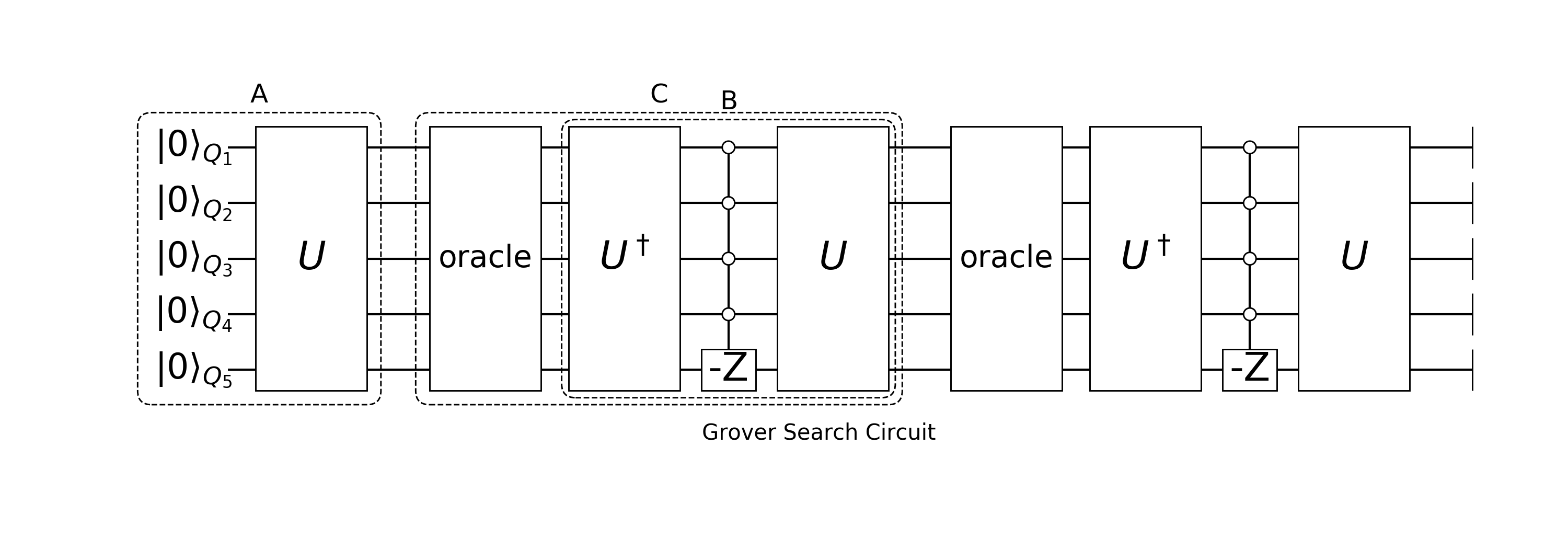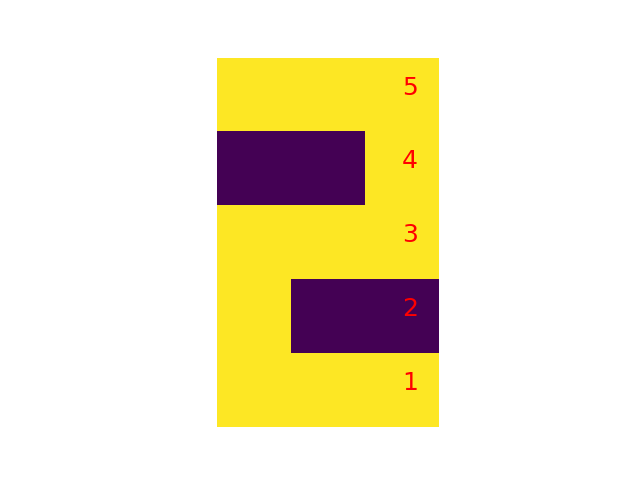Grover Search and Quantum Inference

Grover Search and Quantum Inference

Grover SearchFirst, we construct the reflection block $R(|\psi\rangle) = 2|\psi\rangle\langle\psi|-1$, given we know how to construct $|\psi\rangle=A|0\rangle$. Then it equivalent to construct $R(|\psi\rangle) = A(2|0\rangle\langle 0|-1)A^\dagger$

using Yao
using Yao.Blocks
using Test, LinearAlgebra
using StatsBase

"""
A way to construct oracle, e.g. inference_oracle([1,2,-3,5]) will
invert the sign when a qubit configuration matches: 1=>1, 2=>1, 3=>0, 5=>1.
"""
function inference_oracle(locs::Vector{Int})
control(locs[1:end-1], abs(locs[end]) => (locs[end]>0 ? Z : chain(phase(π), Z)))
end

function reflectblock(A::MatrixBlock{N}) where N
chain(N, A |> adjoint, inference_oracle(-collect(1:N)), A)
end

nbit = 12
A = repeat(nbit, H)
ref = reflectblock(A)

@testset "test reflect" begin
reg = rand_state(nbit)
ref_vec = apply!(zero_state(nbit), A) |> statevec
v0 = reg |> statevec
@test -2*(ref_vec'*v0)*ref_vec + v0 ≈ apply!(copy(reg), ref) |> statevec
end

Then we define the oracle and target state

# first, construct the oracle with desired state in the range 100-105.
oracle!(reg::DefaultRegister) = (reg.state[100:105,:]*=-1; reg)

# transform it into a function block, so it can be put inside a Sequential.
fb_oracle = FunctionBlock{:Oracle}(reg->oracle!(reg))

"""
ratio of components in a wavefunction that flip sign under oracle.
"""
function prob_match_oracle(psi::DefaultRegister, oracle)
fliped_reg = apply!(register(ones(ComplexF64, 1<<nqubits(psi))), oracle)
match_mask = fliped_reg |> statevec |> real .< 0
end

# uniform state as initial state
psi0 = apply!(zero_state(nbit), A)

# the number of grover steps that can make it reach first maximum overlap.
num_grover_step(prob::Real) = Int(round(pi/4/sqrt(prob)))-1
niter = num_grover_step(prob_match_oracle(psi0, fb_oracle))

# construct the whole circuit
gb = sequence(sequence(fb_oracle, ref) for i = 1:niter);

Now, let's start training

for (i, blk) in enumerate(gb)
apply!(psi0, blk)
overlap = prob_match_oracle(psi0, fb_oracle)
println("step $i, overlap =$overlap")
end

The above is the standard Grover Search algorithm, it can find target state in $O(\sqrt N)$ time, with $N$ the size of an unordered database. Similar algorithm can be used in more useful applications, like inference, i.e. get conditional probability distribution $p(x|y)$ given $p(x, y)$.

function rand_circuit(nbit::Int, ngate::Int)
circuit = chain(nbit)
gate_list = [X, H, Ry(0.3), CNOT]
for i = 1:ngate
gate = rand(gate_list)
push!(circuit, put(nbit, (sample(1:nbit, nqubits(gate),replace=false)...,)=>gate))
end
circuit
end
A = rand_circuit(nbit, 200)
psi0 = apply!(zero_state(nbit), A)

# now we want to search the subspace with [1,3,5,8,9,11,12]
# fixed to 1 and [4,6] fixed to 0.
evidense = [1, 3, -4, 5, -6, 8, 9, 11, 12]

"""
Doing Inference, psi is the initial state,
the target is to search target space with specific evidense.
e.g. evidense [1, -3, 6] means the [1, 3, 6]-th bits take value [1, 0, 1].
"""
oracle_infer = inference_oracle(evidense)(nqubits(psi0))

niter = num_grover_step(prob_match_oracle(psi0, oracle_infer))
gb_infer = chain(nbit, chain(oracle_infer, reflectblock(A)) for i = 1:niter);

Now, let's start training

for (i, blk) in enumerate(gb_infer)
apply!(psi0, blk)
p_target = prob_match_oracle(psi0, oracle_infer)
println("step $i, overlap^2 =$p_target")
end

Here is an application, suppose we have constructed some digits and stored it in a wave vector.

using Yao.Intrinsics

x1 = [0 1 0; 0 1 0; 0 1 0; 0 1 0; 0 1 0]
x2 = [1 1 1; 0 0 1; 1 1 1; 1 0 0; 1 1 1]
x0 = [1 1 1; 1 0 1; 1 0 1; 1 0 1; 1 1 1]

nbit = 15
v = zeros(1<<nbit)

# they occur with different probabilities.
for (x, p) in [(x0, 0.7), (x1, 0.29), (x2,0.01)]
v[(x |> vec |> BitArray |> packbits)+1] = sqrt(p)
end

Plot them, you will see these digitsThen we construct the inference circuit. Here, we choose to use reflect to construct a ReflectBlock, instead of constructing it explicitly.

rb = reflect(copy(v))
psi0 = register(v)

# we want to find the digits with the first 5 qubits [1, 0, 1, 1, 1].
evidense = [1, -2, 3, 4, 5]
oracle_infer = inference_oracle(evidense)(nbit)

niter = num_grover_step(prob_match_oracle(psi0, oracle_infer))
gb_infer = chain(nbit, chain(oracle_infer, rb) for i = 1:niter)

Now, let's start training

for (i, blk) in enumerate(gb_infer)
apply!(psi0, blk)
p_target = prob_match_oracle(psi0, oracle_infer)
println("step $i, overlap^2 =$p_target")
end

The result is

pl = psi0 |> probs
config = findfirst(pi->pi>0.5, pl) - 1 |> bitarray(nbit)
res = reshape(config, 5,3)
5×3 BitArray{2}:
true   true  true
true  false  true
true  false  true
true  false  true
true   true  true

It is 2 ~Congratuations! You get state of art quantum inference circuit!